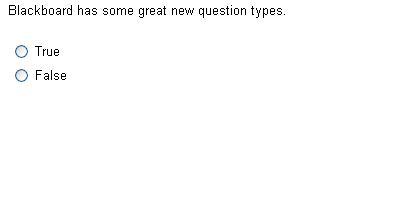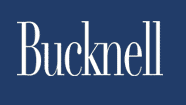## New Question Types in Blackboard 6.3

#### January 3, 2006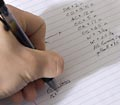With the upgrade to version 6.3 of Blackboard, there are a number of new questions types that can be used in tests and surveys: Calculated Numeric; Calculated Formula; Either/Or; File Response; Fill in Multiple Blanks; Hot Spot; Jumbled Sentence; Opinion Scale/Likert; Quiz Bowl; Short Answer. The original questions types -- Essay; Fill in the Blank; Matching; Multiple Answer; Multiple Choice; Ordering; True/False-- are still available.

Calculated Numeric

This question type is similar to a fill-in-the blank question, except that the answer (1) is evaluated as numeric (not just text), and you can specify an answer range. For example, if the correct answer is .1, then .1, 0.1, and .10 would all be accepted as correct answers. And if you specified a range of ± .01, then .11 and .09 would also be accepted as correct.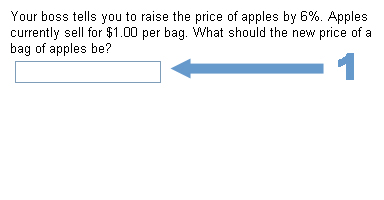Calculated Formula

In a Calculated Formula question, the numeric values used in the question (1) can be different for each person taking a quiz (or each time a person takes the quiz if you allow multiple attempts). You specify allowable ranges for each variable. The correct answer (2) is calculated from the actual numeric values used for a particular question, based on the formula that you provide. Like the Calculated Numeric questions, the answer is evaluated as a numeric (not text), and you can specify an acceptable range.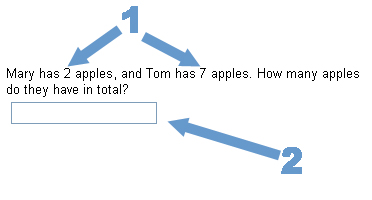Either/Or

The Either/Or question is similar to a True/False question, except that you have several options for the answer label (1): Yes/No; Agree/Disagree; Right/Wrong; True/False. The answer choices can be organized horizontally (as illustrated) or vertically.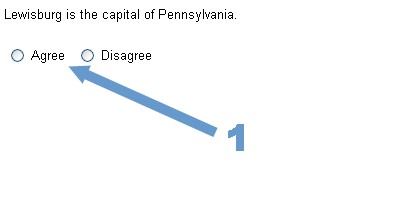File Response

To answer a File Response question, the student hands in a file (1) from their local computer (or file services space). It might be a Word document that they created in response to an essay question, or it could be an Excel spreadsheet, for example.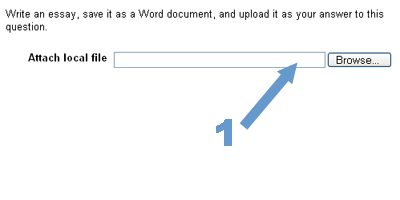Fill in Multiple Blanks

The Fill in Multiple Blanks question is the same as a Fill in the Blank question, except that you can have more than one blank in the question. You can give partial credit, or require that all blanks be filled correctly to get any credit. Note that the 'blanks' are embedded within the question text.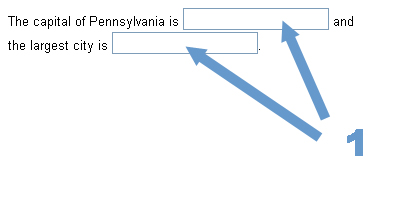Hot Spot

In a Hot Spot question, the student answers by clicking on a particular location within an image (1). When setting up the question, you determine what area (a rectangle) within the image is 'correct' (2).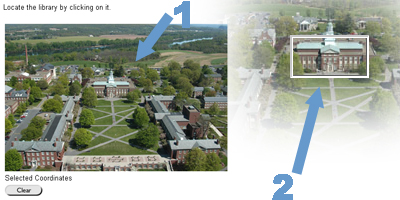Jumbled Sentence

The Jumbled Sentence question is similar to the Fill in Multiple Blanks question, except that there is a drop-down box (1) for each blank. (Note that it is the same set of choices for any blanks within the same question.)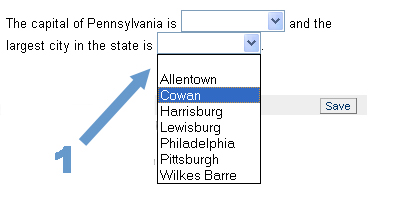Opinion Scale/Likert

Opinion Scale/Likert questions are probably more useful for surveys than tests, but you can specify a correct answer if you are using them in a test. The choices (1) default to Strongly Agree, Agree, Neither Agree nor Disagree, Disagree, Strongly Disagree, Not Applicable, but you can change them to anything that you want.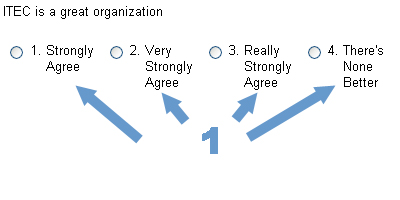Quiz Bowl

Think Jeopardy. Answers must be in the form of a question. [This may be fairly complicated to set up so that it gets graded correctly.]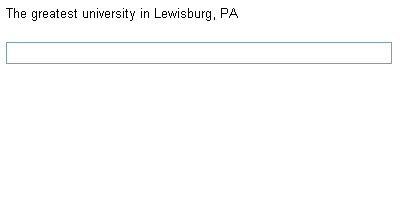The Short Answer question is the same as an essay question, except that you specify the size (1) of the answer box to suggest (though not enforce) the appropriate length of the answer.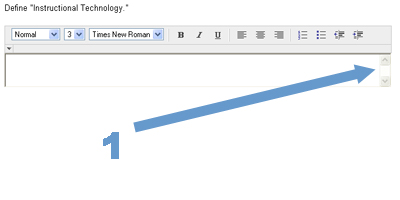The original question types are still available.

Essay

The Essay question is used for any type of text-based answer. It cannot be automatically graded by Blackboard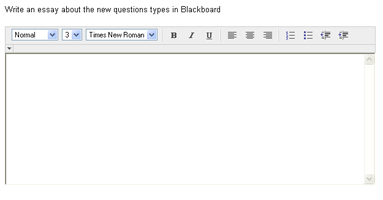Fill in the Blank

The Fill in the Blank question is used for short answers (1): a single word or short phrase. The response must exactly match the answer, including spelling, to get this correct.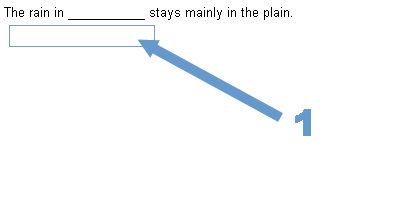Matching

For Matching questions, you can allow partial credit, or not. You can have choices displayed in random order, and each answer can be labeled with Arabic (as illustrated) or Roman numerals, or letters.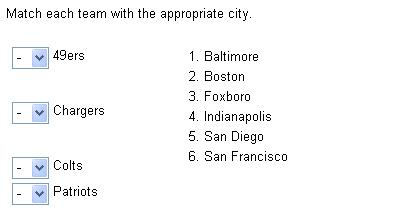For Multiple Answer questions, the answers can be arranged vertically or horizontally (as illustrated). They can be in random order, You can allow partial credit, or not.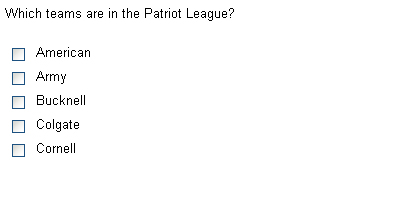Multiple Choice

Similar to Multiple Answer, the Multiple Choice question has options for orientation, order, partial credit, and numbering.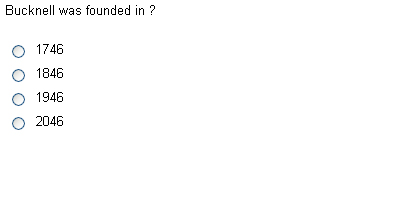Ordering

For Ordering questions, there are now options for partial credit and numbering of answers.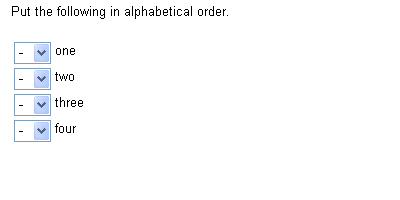True/False

The classic True/False question.